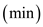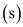# What is the difference between a dimension and a unit? Give three examples of each.

Question-AnswerCategory: Fluid MechanicsWhat is the difference between a dimension and a unit? Give three examples of each.

What is the difference between a dimension and a unit? Give three examples of each.

A unit is a way of assigning a number to dimension. Units have different systems of notations such as SI system, M.K.S system, F.P.S system etc. For example, time is a dimension that is measured in days, hours, minutes, seconds, millisecondsetc.